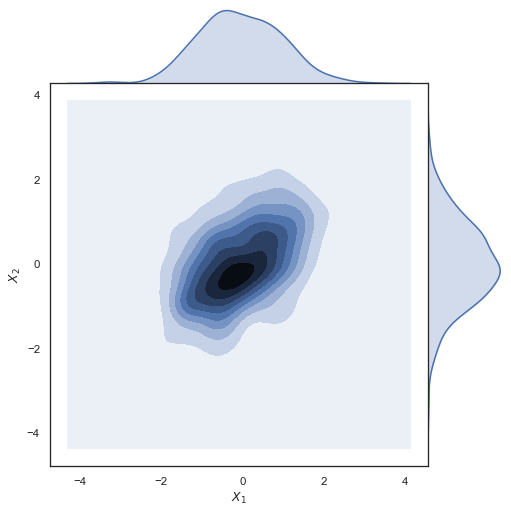# Joint kernel density estimate¶Python source code: `[download source: joint_kde.py]`

```import numpy as np
import pandas as pd
import seaborn as sns
sns.set(style="white")

# Generate a random correlated bivariate dataset
rs = np.random.RandomState(5)
mean = [0, 0]
cov = [(1, .5), (.5, 1)]
x1, x2 = rs.multivariate_normal(mean, cov, 500).T
x1 = pd.Series(x1, name="\$X_1\$")
x2 = pd.Series(x2, name="\$X_2\$")

# Show the joint distribution using kernel density estimation
g = sns.jointplot(x1, x2, kind="kde", height=7, space=0)
```